## Exercises

1.
For the following functions and base points, determine the smallest order that is required so that the Taylor polynomial approximates the function to within a tolerance of 0.05 over the given interval. Be sure to explain how you got your answers in your report. This means including any plots that you used to make your conclusion.
(a)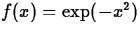, a=0, interval [-1,1].
(b), a=0, interval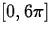.
(c)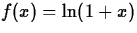, a=0, interval [-0.9,0.9].
(d)
f(x) = 1/x, a=1, interval [0.5, 3].
2.
Use the Taylor polynomial to approximate the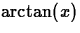at a=0, choose any n you want. Determine the general formula for the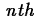term of the polynomial. Write the polynomial as a finite series and then evaluating the series at x=1 find the value of n that will calculateto four decimal-place accuracy.

3.
For the third function in exercise 1,, consider the Taylor polynomial about a=0. Can you choose the order so that the Taylor polynomial is a good approximation (within 0.1, say) toat x=2? How about at x=0.5? Discuss the difference in the behavior of the Taylor polynomials at these two points. Can you divide the real line up into two parts, one where the approximation is good and one where it is bad?Next: About this document ... Up: Taylor Polynomials Previous: Accuracy and Tolerance

Christine M Palmer
11/21/1997